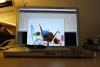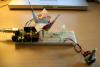# Theory and Practice of Tangible User Interfaces

### Announcements

November 24, 2007
Reading for November 27th, are now posted. Enjoy!

October 2, 2007
To upload your thoughtless acts, create a new assignment page like any other lab. You'll see "Thoughtless Acts" listed as one of the assignment options.

May 24, 2008
This site has been archived and is no longer editable. Stay tuned for the next version, coming in the fall!

# ThirdSqueeze

Project Members:
Ken-ichi Ueda

## Description

My original intent was to make squeezing the FSR squeeze a sort of pastey goo on the screen, but all I was able to get was connected bouncing balls. They way it works now is that when you squeeze the back of the origami crane mounted on the breadboard, strings of connected balls begin falling onto the screen. You can use the pot to control their initial direction.

## Components

• Pot
• FSR
• LEDs
• Origami crane

## Arduino Code

`int fsrPin = 0;  // select the input pin for the sensor`
`int potPin = 1;`
`int bledPin = 11;    // select the output pin for the LED`
`int gledPin = 10;`
`int fsrVal = 0;        // variable to store the value coming from the sensor`
`int potVal = 0;`
`void setup() {`
`  Serial.begin(9600);`
`}`
`void loop() {`
`  fsrVal = analogRead(fsrPin); // read the value from the sensor, 0-1023`
`  potVal = analogRead(potPin);`
`  analogWrite(bledPin, fsrVal/4);  // analogWrite (dimming the LED) can be between 0-255`
`  analogWrite(gledPin, potVal/4);`
`  Serial.print("f");`
`  Serial.println(fsrVal/4);       // writing the value to the PC via serial connection`
`  Serial.print("p");`
`  Serial.println(potVal/4);       // writing the value to the PC via serial connection  `
`  delay(50);                   // rest a little...`
`}`

## Processing Code

`import java.util.Vector;`
`import processing.serial.*;`
`String portname = "/dev/tty.usbserial-A4001nLM";`
`Serial port;`
`String buf="";`
`int cr = 13;  // ASCII return   == 13`
`int lf = 10;  // ASCII linefeed == 10`
`int cFsrVal = 0;`
`int pFsrVal = 0;`
`int cPotVal = 0;`
`int pPotVal = 0;`
`char dev; // current device ID from serial ('f' == FSR, 'p' == POT)`
`int val; // current device value from serial`
`int numBalls = 12;`
`float spring = 0.9;`
`float gravity = 2;`
`int WINWIDTH = 800;`
`int WINHEIGHT = 600;`
`GoopString cGoop;`
`Vector goops = new Vector();`
`GoopString iGoop; // for iterating`
`int i = 0; // iterator`
`int maxLength = 5;`
`void setup() {`
`  size(WINWIDTH, WINHEIGHT);`
`  // frameRate(10);`
`  noStroke();`
`  smooth();`
`  background(0); // redraw bg?`
`  port = new Serial(this, portname, 9600);`
`}`
`void draw() {`
`  // draw stuff`
`  background(#D4EFFD);`
`  // draw the goop`
`  print("# of goops: ");`
`  println(goops.size());`
`  if (goops.size() > 0) {`
`    if (frameCount % 20 == 0 && cGoop.balls.size() < maxLength) {`
`      cGoop.grow();`
`    }`
`    for (int j = 0; j < goops.size(); j++) {`
`      print("Drawing goop #");`
`      println(j);`
`      iGoop = (GoopString) goops.get(j);`
`      iGoop.move();`
`      iGoop.display();`
`    }`
`  }`
`}`
`void keyPressed() {`
`  if (key == ' ') {`
`    println("Wiping the screen...");`
`    background(0);`
`    goops.removeAllElements();`
`  }`
`  else {`
`    makeGoop(40.0);`
`  }`
`}`
`void makeGoop(float din) {`
`  color tClr = color(random(255), random(255), random(255));`
`  goops.addElement(new GoopString(width / 2, 0, cPotVal, 0.0, din, tClr));`
`  cGoop = (GoopString) goops.lastElement();`
`}`
`// called whenever serial data arrives`
`void serialEvent(Serial p) {`
`  int c = port.read();`
`  if (c != lf && c != cr) {`
`    buf += char(c);`
`  }`
`  if (c == lf) {`
`    dev = buf.charAt(0);`
`    val = int(buf.substring(1));`
`    println("dev="+dev+", val="+val);`
`    if (dev == 'f') {`
`      pFsrVal = cFsrVal;`
`      cFsrVal = val;`
`      if (cFsrVal > 5) {`
`        makeGoop(cFsrVal);`
`      }`
`    }`
`    else if (dev == 'p') {`
`      pPotVal = cPotVal;`
`      cPotVal = (int) (val / 6.375 - 20); // get pot val in -20 to 20 range`
`    }`
`    buf = "";`
`  }`
`}`
`// roughly adapted from the Chains example`
`class GoopString {`
`  float x, y;`
`  float diameter;`
`  float vx, vy;`
`  color clr;`
`  Vector balls = new Vector();`
`  Ball cBall; // current ball in a loop`
`  Ball pBall; // prev ball in a loop`
`  `
`  GoopString(float xin, float yin, float vxin, float vyin, float din) {`
`    x = xin;`
`    y = yin;`
`    vx = vxin;`
`    vy = vyin;`
`    diameter = din;`
`    clr = #000000;`
`    balls.addElement(new Ball(xin, yin, vxin, vyin, din, clr));`
`  }`
`  GoopString(float xin, float yin, float vxin, float vyin, float din, color clrin) {`
`    x = xin;`
`    y = yin;`
`    vx = vxin;`
`    vy = vyin;`
`    diameter = din;`
`    clr = clrin;`
`    balls.addElement(new Ball(xin, yin, vxin, vyin, din, clr));`
`  }`
`  `
`  // add a new node onto the GoopString`
`  void grow() {`
`    cBall = new Ball(x, y, vx, vy, diameter);`
`    balls.addElement(cBall);`
`  }`
`  `
`  void move() {`
`    for (i = 0; i < balls.size(); i++) {`
`      cBall = (Ball) balls.get(i);`
`      cBall.move();`
`    }`
`  }`
`  `
`  void display() {`
`    for (i = 0; i < balls.size(); i++) {`
`      cBall = (Ball) balls.get(i);`
`      cBall.display();`
`      if (i > 0) {`
`        stroke(clr);`
`        strokeWeight(diameter);`
`        pBall = (Ball) balls.get(i-1);`
`        line(cBall.x, cBall.y, pBall.x, pBall.y);`
`      }`
`    }`
`  }`
`  `
`}`
`// Ball class adapted from the BouncyBubbles example`
`class Ball {`
`  float x, y;`
`  float diameter;`
`  float vx = 0;`
`  float vy = 0;`
`  color clr;`
` `
`  Ball(float xin, float yin, float vxin, float vyin, float din) {`
`    x = xin;`
`    y = yin;`
`    diameter = din;`
`    vx = vxin;`
`    vy = vyin;`
`    clr = #ffffff;`
`  }`
`  `
`  Ball(float xin, float yin, float vxin, float vyin, float din, color clrin) {`
`    x = xin;`
`    y = yin;`
`    diameter = din;`
`    vx = vxin;`
`    vy = vyin;`
`    clr = clrin;`
`  }`
`  `
`  void move() {`
`    //vx *= 0.999;`
`    vy += gravity;`
`    x += vx;`
`    y += vy;`
`    if (x + diameter/2 > width) {`
`      x = width - diameter/2;`
`      vx += -0.9; `
`    }`
`    else if (x - diameter/2 < 0) {`
`      x = diameter/2;`
`      vx *= -0.9;`
`    }`
`    if (y + diameter/2 > height) {`
`      y = height - diameter/2;`
`      vy *= -spring; `
`    } `
`    else if (y - diameter/2 < 0) {`
`      y = diameter/2;`
`      vy *= -spring;`
`    }`
`  }`
`  `
`  void display() {`
`    noStroke();`
`    fill(clr);`
`    ellipse(x, y, diameter, diameter);`
`  }`
`}`

## PicturesThirdSqueeze: Board & ScreenThirdSqueeze: More of the Board